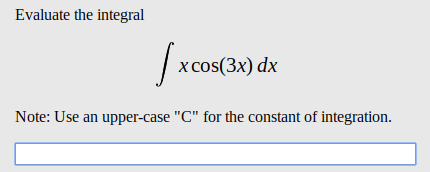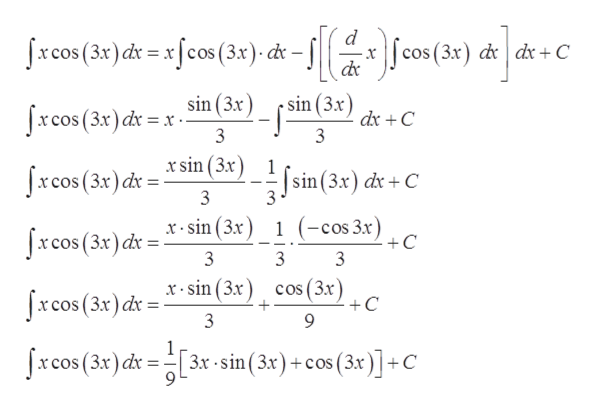# Evaluate the integralx cos(3x) dxNote: Use an upper-case "C" for the constant of integration

Question
11 viewshelp_outlineImage TranscriptioncloseEvaluate the integral x cos(3x) dx Note: Use an upper-case "C" for the constant of integration fullscreen
check_circle

Step 1

Given:

Step 2

Integration by parts:

Step 3

Taking x as first function and cos(3x) as second function and I...help_outlineImage Transcriptionclosed Jxcos (3x) dr= xfcos(3x) d cos(3x) d dx+C x sin (3x xcos (3x) dr= x sin (3x dx C xsin (3x) Jxcos (3x) dx= sin(3x) dx+ C x sin (3x) (-cos 3x) +C 1 Jxcos (3x) dr= 3 x sin (3x) cos ( 3.x) +C 9 Jxcos (3x) dr= 3 Jxcos (3x) dt 3x sin(3x)+cos (3x)+C fullscreen

### Want to see the full answer?

See Solution

#### Want to see this answer and more?

Solutions are written by subject experts who are available 24/7. Questions are typically answered within 1 hour.*

See Solution
*Response times may vary by subject and question.
Tagged in

### Calculus